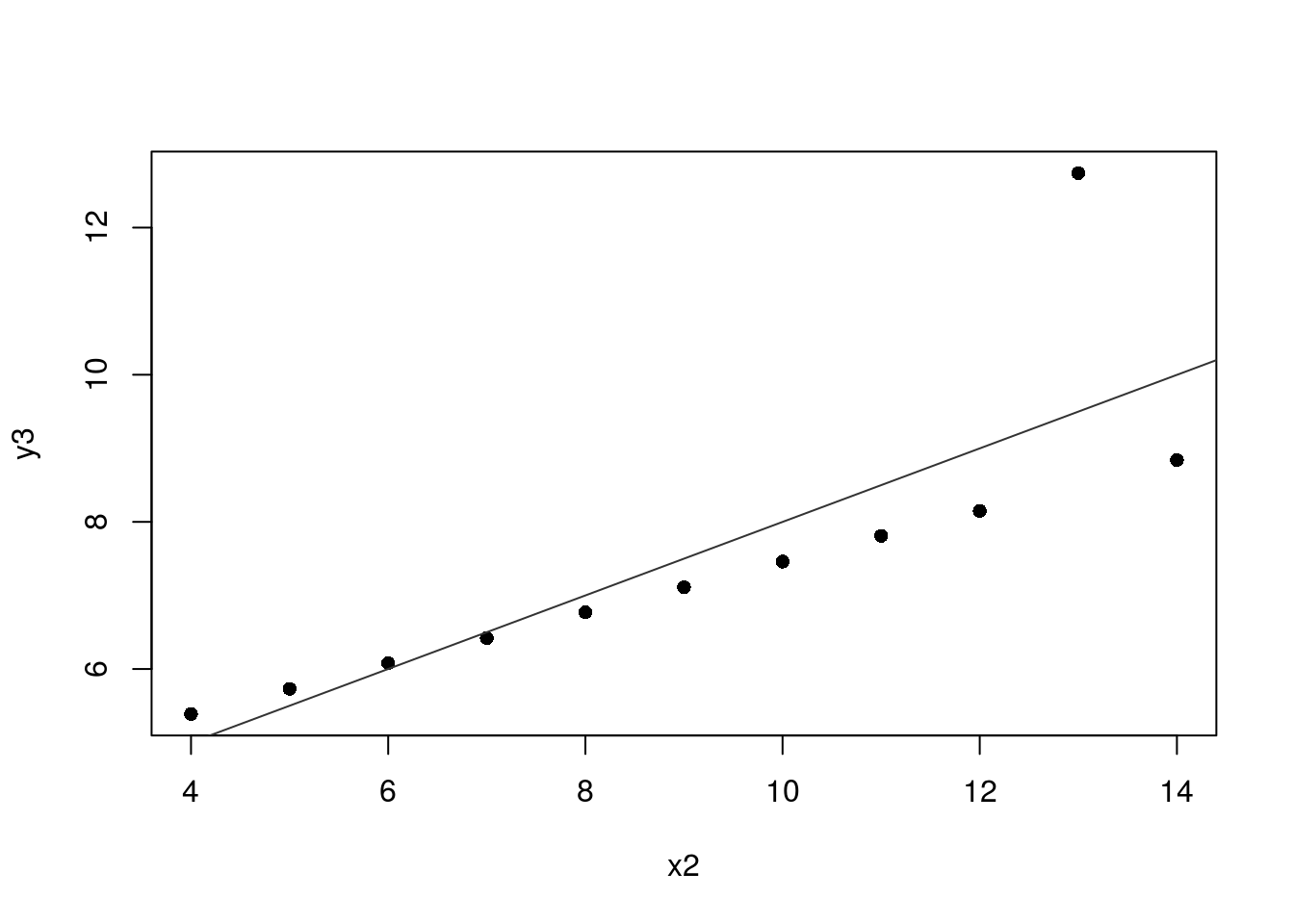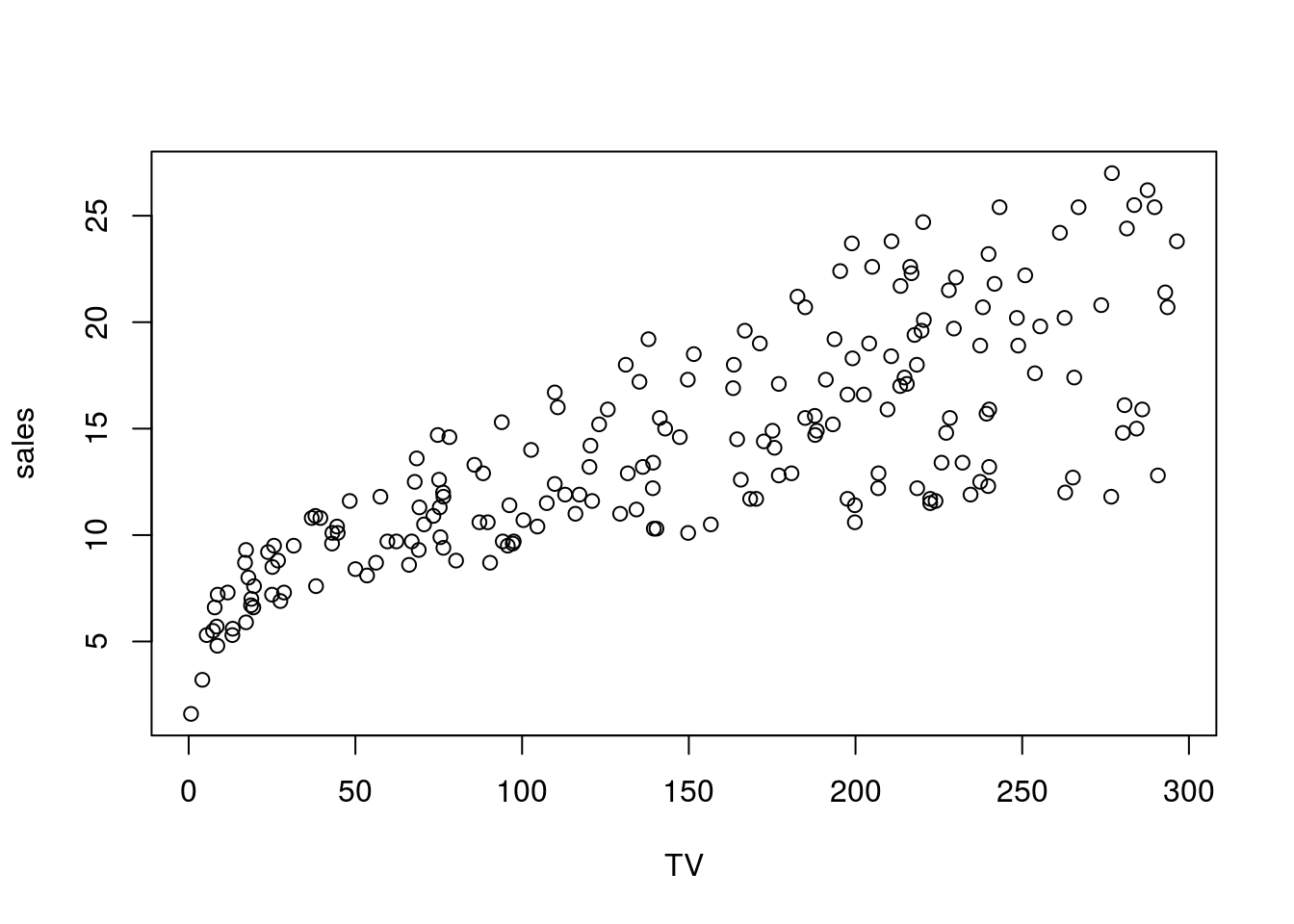## R Markdown

This is an R Markdown document. Markdown is a simple formatting syntax for authoring HTML, PDF, and MS Word documents. For more details on using R Markdown see http://rmarkdown.rstudio.com.

Note: this analysis was performed using the open source software R and Rstudio.

## Objective

The objective of this tutorial is to explain how bivariate analysis works.This analysis can be used by marketers to make decisions about their pricing strategies, advertising strategies, and promotion stratgies among others.

Bivariate analysis is one of the simplest forms of statistical analysis. It is generally used to find out if there is a relationship between two sets of values (or two variables). That said, it usually involves the variables X and Y (statisticshowto.com).

• Univariate analysis is the analysis of one (“uni”) variable.
• Bivariate analysis is the analysis of exactly two variables.
• Multivariate analysis is the analysis of more than two variables.

## Dataset - We will be using two online datasets available in R for this tutorial

plot(y3 ~ x2, data = anscombe, pch = 16)
abline(lm(y3 ~ x3, anscombe), col = "grey20")### Question 1:is there a relationship between x and y? If so, how does the relationship look like?

The relationship is positive

library(readr)
ad_sales <- read_csv('https://raw.githubusercontent.com/utjimmyx/regression/master/advertising.csv')
## Warning: Missing column names filled in: 'X1' 
## Warning: Duplicated column names deduplicated: 'X1' => 'X1_1' 
##
## ── Column specification ────────────────────────────────────────────────────────
## cols(
##   X1 = col_double(),
##   X1_1 = col_double(),
##   TV = col_double(),
##   newspaper = col_double(),
##   sales = col_double()
## )
plot(sales ~ TV, data = ad_sales)